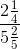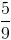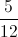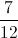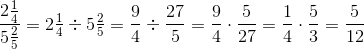# SSAT Upper Level Math : How to find a complex fraction

## Example Questions

### Example Question #1 : How To Find A Complex Fraction

Simplify: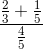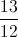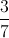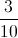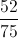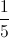Explanation:

Add the fractions in the numerator, then divide the sum by the denominator: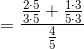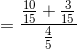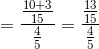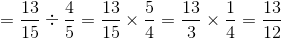### Example Question #2 : How To Find A Complex Fraction

Simplify: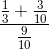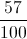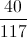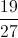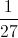Explanation:

Simplify the sum in the numerator, then divide by the denominator: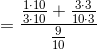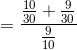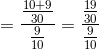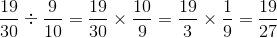### Example Question #1 : How To Find A Complex Fraction

Simplify: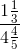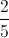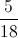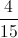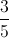Explanation:

Rewrite this as a division problem, then solve: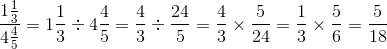### Example Question #4 : How To Find A Complex Fraction

Simplify: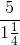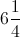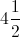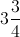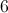Explanation:

Rewrite this as a division problem, then solve: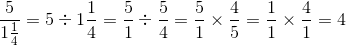### Example Question #5 : How To Find A Complex Fraction

Simplify: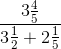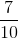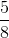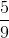Explanation: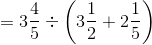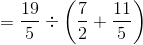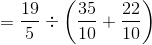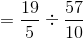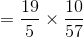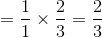### Example Question #6 : How To Find A Complex Fraction

Simplify: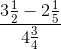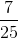The correct answer is not given among the other responses.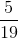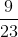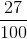The correct answer is not given among the other responses.

Explanation: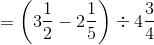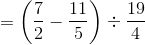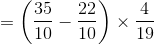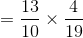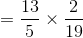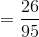This is not among the given responses.

### Example Question #7 : How To Find A Complex Fraction

Simplify: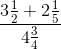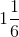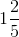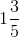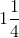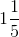Explanation: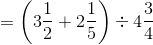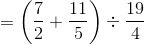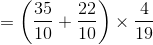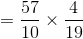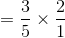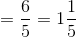### Example Question #2 : How To Find A Complex Fraction

Simplify, writing as a proper fraction.